﻿ 基于混合优化算法的船舶分段堆场间调度研究
«上一篇文章快速检索 高级检索

 哈尔滨工程大学学报2018, Vol. 39Issue (12): 2025-2032  DOI: 10.11990/jheu.2017060710

### 引用本文LI Baihe, JIANG Zuhua, TAO Ningrong, et al. Research on dispatch of blocks between stockyards based on hybrid optimization algorithm[J]. Journal of Harbin Engineering University, 2018, 39(12), 2025-2032. DOI: 10.11990/jheu.201706071.### 文章历史

1. 上海交通大学 机械与动力工程学院, 上海 200240;
2. 高新船舶与深海开发装备协同创新中心, 上海, 200240;
3. 上海海洋大学 工程学院, 上海 201306

Research on dispatch of blocks between stockyards based on hybrid optimization algorithm
LI Baihe 1,2, JIANG Zuhua 1,2, TAO Ningrong 3, MENG Lingtong 1,2
1. School of Mechanical Engineering, Shanghai Jiao Tong University, Shanghai 200240, China;
2. Collaborative Innovation Center for Advanced Ship and Deep-Sea Exploration, Shanghai 200240, China;
3. College of Engineering Science and Technology, Shanghai Ocean University, Shanghai 201306, China
Abstract: To realize an effective dispatch scheme of transportation flatcars in shipyards, a model was developed based on the constraints of flatcars load capacity, tasks priority, time window of tasks, and the running time of unloaded flatcars. The penalty weight resulting from the time window constraints was used as a model of the optimization goal. A good feasible solution was obtained by a genetic algorithm, and then Tabu search algorithm was used for constructing two kinds of neighborhood spatial strategy to solve the optimal sequence of the carriage blocks. The depth-first traversal algorithm was used to solve the optimal path considering the number of turns, so as to achieve solution to the model. Finally, the application of the model was verified using the actual data of a shipyard. The results show that the proposed method can solve the optimal scheduling scheme and result in efficient use of resources.
Keywords: dispatch between storage yards    multi-type flatcars    prior constraint of tasks    time window constraints    genetic algorithm    Tabu search algorithm    two kinds of neighborhood spatial strategy    depth-first search

1 堆场间调度问题描述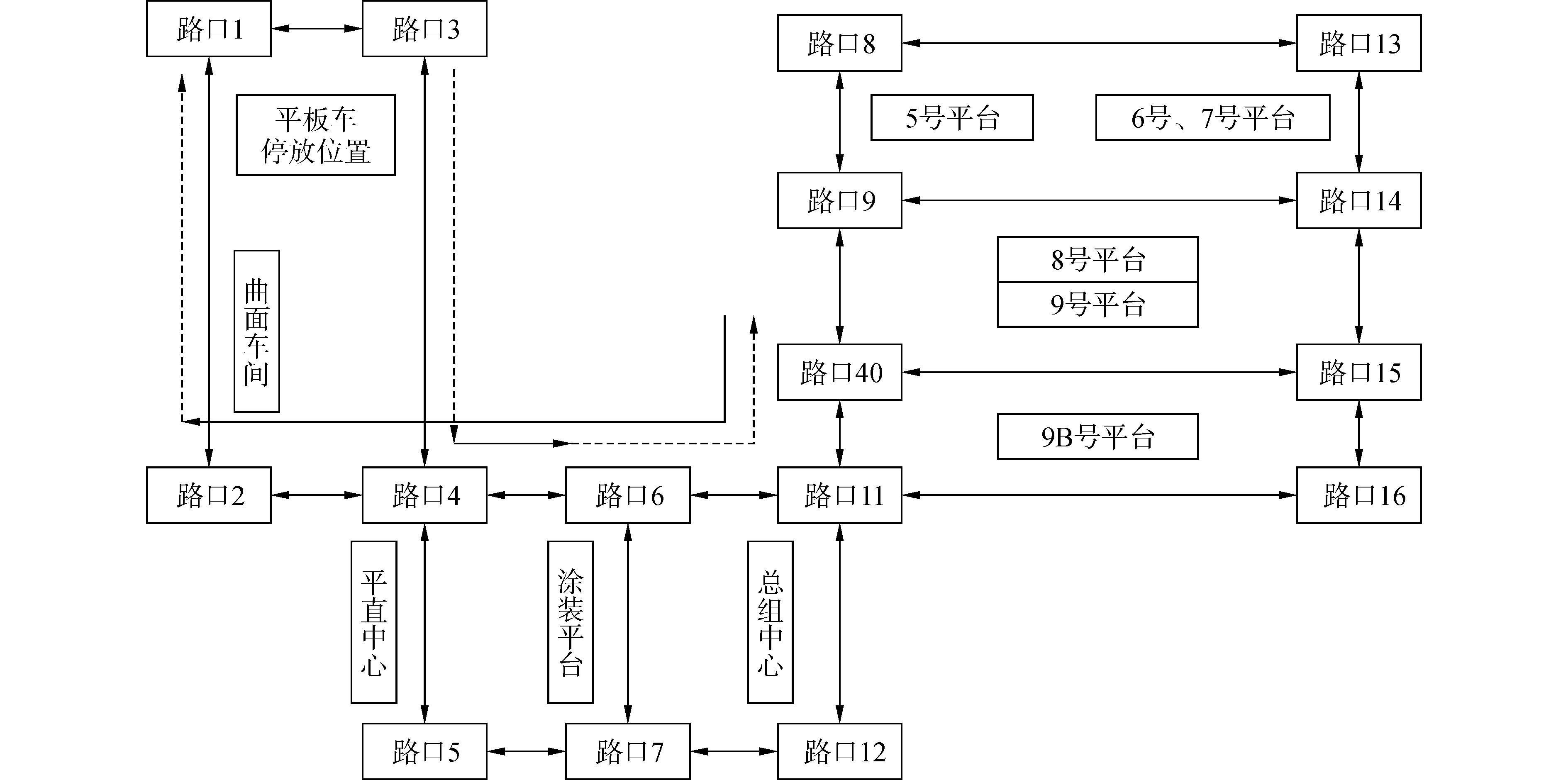Download: 图 1 道路及堆场分布图 Fig. 1 Roads and yards distribution

2 堆场间调度模型的建立 2.1 堆场路口矩阵图

 $\mathit{\boldsymbol{H}} = \left[ {\begin{array}{*{20}{c}} {{H_{11}}}& \cdots &{{H_{116}}}\\ \vdots & \ddots & \vdots \\ {{H_{161}}}& \cdots &{{H_{1616}}} \end{array}} \right]$
2.2 平板车运行路径优劣的评价标准

 $\begin{array}{l} \left| {{x_A} - {x_B}} \right| = 0, \left| {{y_B} - {y_C}} \right| = 0, \\ \left| {{y_A} = {y_B}} \right| = 0, \left| {{x_B} - {x_C}} \right| = 0。\end{array}$

 $\begin{array}{l} {X_j} = \left\{ \begin{array}{l} 1, \left| {{x_j} - {x_{j + 1}}} \right| = 0\;\;\;{\rm{and}}\;\;\;\left| {{y_{j + 1}} - {y_{j + 2}}} \right| = 0\\ 0, {\rm{否则}} \end{array} \right., \\ {Y_j} = \left\{ \begin{array}{l} 1, \left| {{y_j} - {y_{j + 1}}} \right| = 0\;\;\;{\rm{and}}\;\;\;\left| {{x_{j + 1}} - {x_{j + 2}}} \right| = 0\\ 0, {\rm{否则}} \end{array} \right., \end{array}$

n为执行任务i时路径中包含的路口个数。

 $f{t_i} + T \times {m_i}$

2.3 堆场间调度模型

1) 决策变量：

yil表示任务i由平板车l执行与否的决策变量。若执行为1，不执行为0。

zihl表示平板车l执行任务顺序的决策变量。若平板车l执行完任务i后紧接着执行任务h为1，否则为0。

zOil表示平板车是否执行其第一个任务的决策变量。若任务i是平板车l的第一个任务则为1，否则为0。

Oi表示任务i是否满足其时间窗始点约束的决策变量。若siri, Oi=0, 若siri, Oi=1。

Ei表示任务i是否满足其时间窗终点约束的决策变量。若cidi, Ei=1，若cidi, Ei=0。其中，ih为运输任务编号，i, h=1, 2, …, nl为平板车编号，l=1, 2, …, msi为任务i的实际开始执行时间；ci为任务i的实际完成时间；ri为任务i的释放时间，即可以开始执行的时间；di为任务i的截止时间，即必须完成的时间。

 $\begin{array}{l} {\rm{Min}}z = \alpha \times \sum\limits_{l = 1}^t {\sum\limits_{h = 1, h \ne i}^n {\sum\limits_{i = 1}^n {{z_{ihl}} \cdot k{t_{ih}}} } } + \\ \beta \times \sum\limits_{i = 1}^n {{O_i} \cdot \left( {{s_i} - {r_i}} \right)} + \gamma \times \sum\limits_{i = 1}^n {{E_i} \cdot \left( {{c_i} - {d_i}} \right)} \end{array}$

2) 约束条件：

 $\sum\limits_{l = 1}^m {{y_{il}} = 1, \;\;\;\;\forall i}$ (1)
 $\left( {{s_i} + f{t_i}} \right) \cdot p{r_{ih}} \le {s_h}, \forall i, h$ (2)
 ${s_i} + f{t_i} + {z_{ihl}} \cdot k{t_{ih}} - {s_h} \le \left( {1 - {z_{ihl}}} \right) \cdot Q, \forall i, h \ne i, l$ (3)
 $C{W_l} \ge {W_i} \cdot {y_{il}}, \forall i, l$ (4)
 $\sum\limits_{i = 1}^n {{z_{Oil}} = 1, \forall l}$ (5)
 $\sum\limits_{j = 1, j \ne i}^n {{z_{jil}} + {z_{Oil}} = {y_{il}}, } \forall i, l$ (6)
 $\sum\limits_{j = 1, j \ne i}^n {{z_{ijl}} \le {y_{il}}, } \forall i, l$ (7)

3 堆场间调度模型求解方法 3.1 模型求解方法

1) 初始化。读取任务信息和平板车信息。

2) 产生初始种群。将待调度任务排序，分配给平板车。形成的一个可行解作为一个调度方案(染色体)。

3) 编码。文中的染色体是基于正整数构成的两个一维数组，分别表示任务序列和平板车序列。任务序列中的第N个基因上的数字表示任务号，平板车序列中的第N个基因上的数字表示执行该任务的平板车号。因此，任务序列和平板车序列是一一对应关系，除此之外，每个车上的任务序列还表示执行顺序。图 2表示10个任务4辆平板车产生的染色体。该染色体表示将任务3分配给平板车1，任务1分配给平板车2，任务5分配给平板车3，任务7分配给平板车4，以此类推。平板车1上有任务3和任务8，那么在平板车执行的时候，先执行任务3再执行任务8。其余平板车同理。Download: 图 2 染色体示例 Fig. 2 Example of the chromosome

4) 解码。计算每个染色体的适应度。即依据任务序列和平板车序列的对应关系将各个任务按照在任务序列中的顺序分配给各个平板车，解码后各个平板车上的任务顺序表示任务执行顺序。依据每个平板车上的任务号调用深度优先遍历算法求解负载运行时间和空载运行时间，得到各个任务的开始执行时间和结束时间。计算适应度。数学模型中的目标函数可以用来对个体表现的评价。但本文中目标函数与个体表现成反比，因此，用一个较大的整数M减去目标函数作为本文中算法的适应度函数。

5) 选择。选择操作使用经典轮盘赌。

6) 单点交叉。两条父代染色体中选择其中一条，随机选择一断点，进行交叉操作。断点右侧基因序列继承到子代时的顺序与另外一个父代中相应基因序列的顺序保持一致，如图 3所示。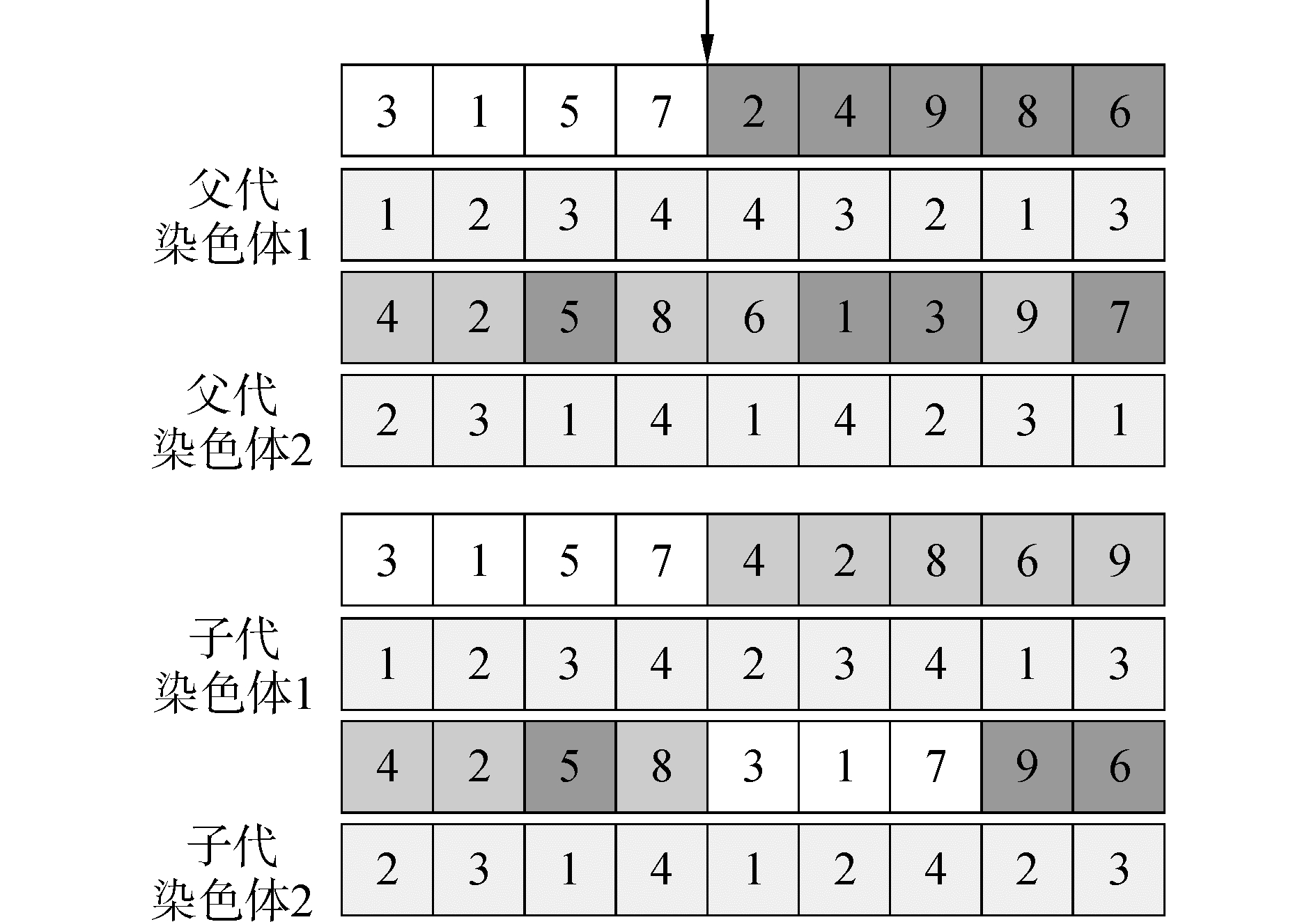Download: 图 3 单点交叉图 Fig. 3 One point crossover

7) 保护约束条件的交换变异。父代染色体中任务之间满足约束条件，因此在进行交换变异中，选择两个点进行交换变异，为了防止因为随机选择破坏任务前后约束关系，设定交换变异的两点选择是在无前后约束的任务中选择。在父代染色体中，假设任务7和任务4是具有前后约束的任务对，那么在随机选择交换变异的任务范围内就不包括任务7和任务4。例如，随机选择结果为任务1和任务9，得到子代的任务序列的过程如图 4所示：在进行交换变异时，需要考虑任务序列变异后的情况是否满足平板车承重能力约束，如果不满足需要随机选择平板车。此时需要判断平板车3和任务1之间是否满足平板车承重与任务重量之间的约束，平板车2和任务9之间同理。如果平板车3和任务1之间不满足约束，则在满足承重要求的平板车里随机产生一个。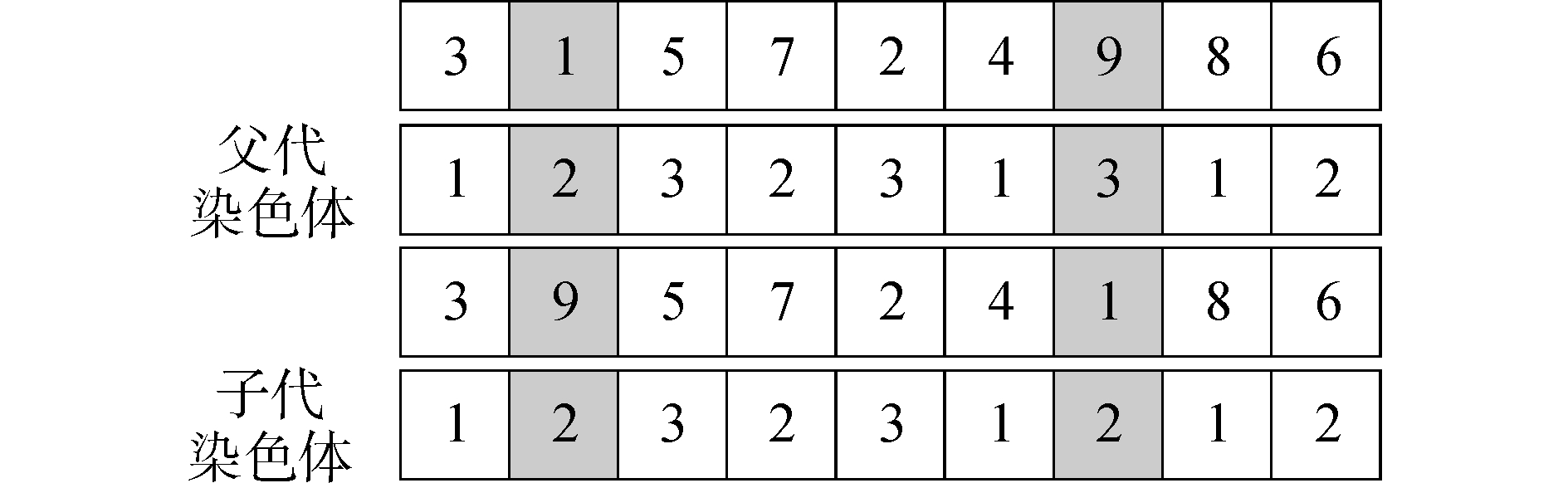Download: 图 4 交换变异 Fig. 4 Exchange mutation

8) 再生。在父代染色体中按适应度大小排列，选择一定数量的适应度高的染色体直接保留到下一代中，即保留父代中“精英”染色体。

9) 禁忌搜索初始化。将遗传算法求得最优序列作为禁忌搜索的初始解。将具有前后任务约束的任务对添加到禁忌表中，并设置其禁忌长度为迭代次数。

10) 禁忌搜索邻域结构。此处设计两种构造邻域解的方法：①对任务序列进行局部搜索。交换两个任务序列的基因获得邻域解，如果交换后不满足平板车承重约束，则随机产生平板车序列；若满足，则不变。②对平板车序列进行局部搜索。选择两个任务序列的基因，随机生成满足其承重要求的平板车。基于以上两种构造邻域解的方法，提出两种策略。

11) 更新禁忌表。禁忌表用于记录邻域变换的操作，进行一次禁忌搜索操作后，更新禁忌长度和禁忌表中的元素。本文采用“任务号-任务号”作为禁忌元素存放在禁忌表中。

12) 特赦准则。当邻域中的所有元素都被禁忌，或者在某次迭代中选择禁忌表中的元素可以得到当前最好的解，则在本次迭代中特赦该禁忌元素。

13) 停止操作。搜索过程中进行两个计数，一个是总迭代步数，另一个是评价函数连续未得到改善的步数。当算法迭代次数达到预先设定的值，将获得的当前个体挑选出来。该个体解码后获得的调度方案即为算法求解的最优方案。

3.2 深度优先遍历算法求解最优路径

1) 读取路口矩阵，构建路口网络图。

2) 读取任务序列，读取任务序列中相应起始堆场内的路径和目标堆场内的路径。

3) 根据坐标求解距离堆位最近的路口start0, end0;start1, end1。

4) 调用深度优先遍历算法求解负载运行和空载运行star0, end0;start1, end1之间的所有可行路径，分别保存在数组sumPath0和sumPath1中。

5) 依据负载运行还是空载运行选择相应的评价函数，计算路径的评价函数值。

6) 计算数组中的所有可行路径，选择评价函数最优的作为最优路径。

4 实例验证与结果分析 4.1 实例验证表 1 堆场间任务计划 Table 1 Task plan between yards表 2 调度方案 Table 2 Dispatch plan

4.2 数值试验分析 4.2.1 算法对比分析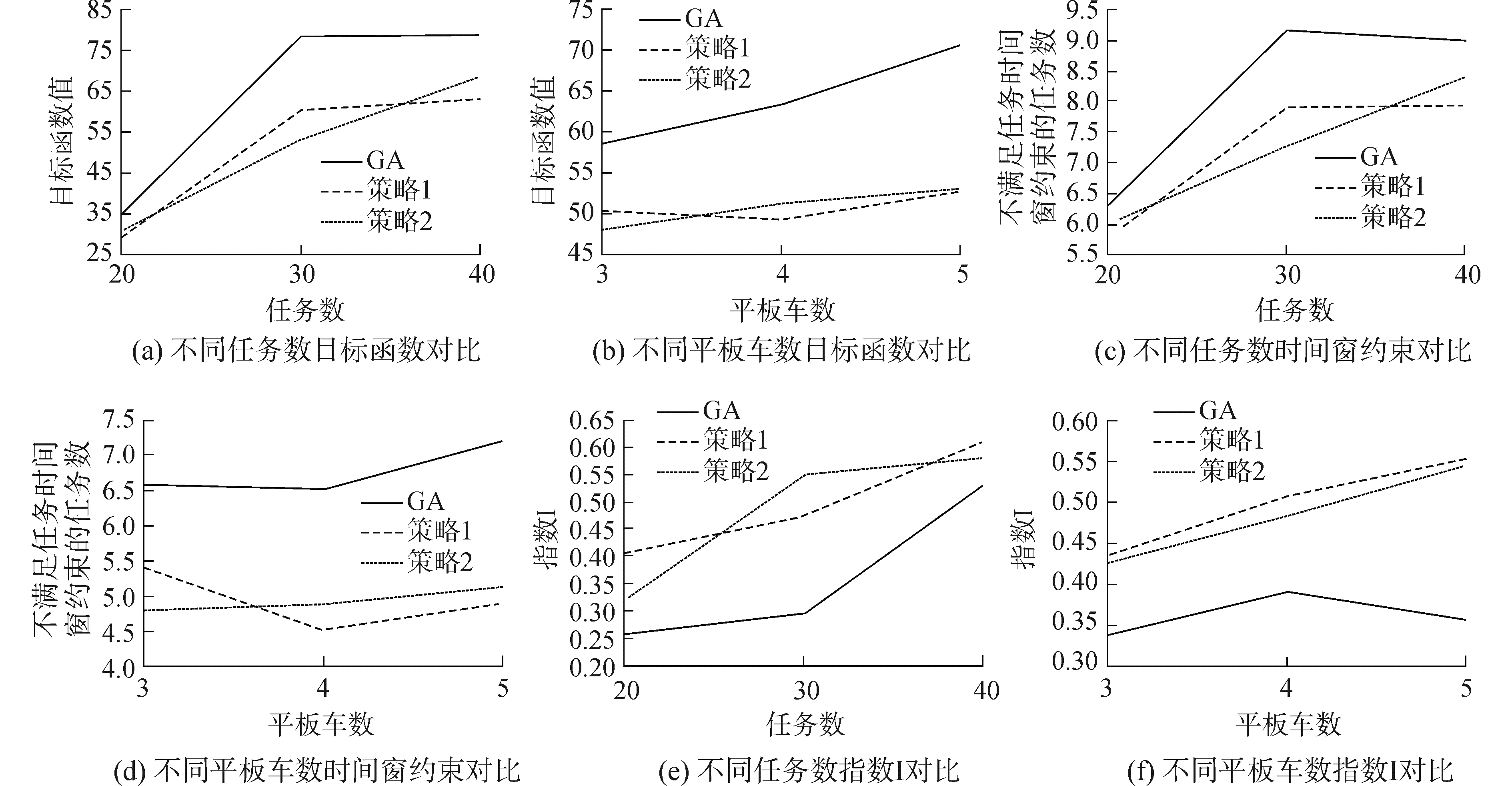Download: 图 5 不同任务数、平板车数求解性能对比 Fig. 5 Contrast about different tasks and flatcars表 3 不同任务数、平板车数求解结果 Table 3 Results about different tasks and flat cars

4.2.2 算法收敛性分析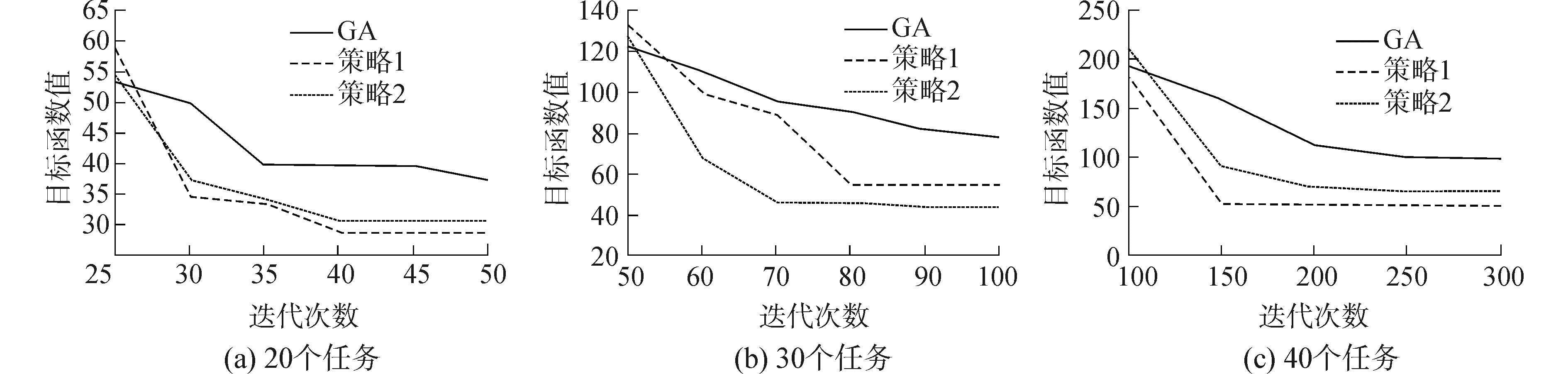Download: 图 6 算法收敛性分析 Fig. 6 Convergence analysis
5 结论

1) 数值实验表明文中提出方法的有效性和实用性, 目标函数相比传统按照调度员经验的分配方式有明显优势，能够解决分段任务数量较大的调度状况。

2) 算法性能方面，相对主流方法，从求解效果和收敛速度方面具有优势。

  ROH M I, CHA J H. A block transportation scheduling system considering a minimisation of travel distance without loading of and interference between multiple transporters[J]. International journal of production research, 2011, 49(11): 3231-3250. DOI:10.1080/00207543.2010.484427 (0)  JOO C M, KIM B S. Block transportation scheduling under delivery restriction in shipyard using meta-heuristic algorithms[J]. Expert systems with applications, 2014, 41(6): 2851-2858. DOI:10.1016/j.eswa.2013.10.020 (0)  张志英, 徐建祥, 计峰. 基于遗传算法的船舶分段堆场调度研究[J]. 上海交通大学学报, 2013, 47(7): 1036-1042. ZHANG Zhiying, XU Jianxiang, JI Feng. Shipbuilding yard scheduling approach based on genetic algorithm[J]. Journal of Shanghai jiao Tong University, 2013, 47(7): 1036-1042. (0)  陶宁蓉.船舶分段建造过程中的资源调度优化研究[D].上海: 上海交通大学, 2013. TAO Ningrong. Research on resouce scheduling problems during ship block assembly process[D]. Shanghai: Shanghai Jiao Tong University, 2013. (0)  张志英, 申钢, 刘祥瑞, 等. 基于最短路算法的船舶分段堆场调度[J]. 计算机集成制造系统, 2012, 18(9): 1982-1990. ZHANG Zhiying, SHEN Gang, LIU Xiangrui, et al. Block storage yard scheduling of shipbuilding based on shortest-path algorithm[J]. Computer integrated manufacturing systems, 2012, 18(9): 1982-1990. (0)  陈凯, 蒋祖华, 刘建峰, 等. 带有进场时间窗的船舶分段堆场调度[J]. 上海交通大学学报, 2016, 50(9): 1390-1398. CHEN Kai, JIANG Zuhua, LIU Jianfeng, et al. Shipbuilding yard scheduling with block inbound time window[J]. Journal of Shanghai jiao Tong University, 2016, 50(9): 1390-1398. (0)  陈云云, 张志英. 船舶分段涂装作业重入调度优化算法[J]. 哈尔滨工程大学学报, 2016, 37(8): 1103-1110. CHEN Yunyun, ZHANG Zhiying. Optimization algorithm for reentrant scheduling in block painting operations[J]. Journal of Harbin Engineering University, 2016, 37(8): 1103-1110. (0)  HU Zhihua, WEI Chen. Synchronizing vehicles for multi-vehicle and one-cargo transportation[J]. Computers & industrial engineering, 2018, 119: 36-49. (0)  曾建智, 张志英. 基于智能算法的船舶分段堆场调度计划与优化[J]. 哈尔滨工程大学学报, 2016, 37(1): 41-47. ZENG Jianzhi, ZHANG Zhiying. Block stockyard scheduling and optimizing based on an intelligent algorithm[J]. Journal of Harbin Engineering University, 2016, 37(1): 41-47. (0)  孟令通, 朱洪渊, 蒋祖华, 等. 基于遗传算法的平板车调度优化方法[J]. 哈尔滨工程大学学报, 2018, 39(3): 554-560. MENG Lingtong, ZHU Hongyuan, JIANG Zuhua, et al. Flat-car scheduling opyimization method based on genetic algorithm[J]. Journal of Harbin Engineering University, 2018, 39(3): 554-560. (0)